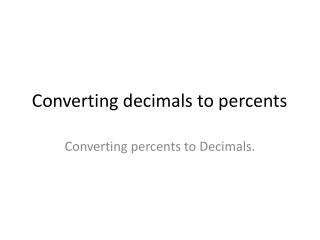DownloadDownload PresentationConverting decimals to percents

# Converting decimals to percents

Télécharger la présentation## Converting decimals to percents

- - - - - - - - - - - - - - - - - - - - - - - - - - - E N D - - - - - - - - - - - - - - - - - - - - - - - - - - -
##### Presentation Transcript

1. Converting decimals to percents Converting percents to Decimals.

2. Changing decimals to percents Move the decimal place 2 positions to the right. .357 = 35.7% remember move 2 to the right. .5 = 50% we have to annex a 0 to have 2 places. 12.678 = 1267.8% - still only 2 places right Your turn now: .45 2.3 .09 .3 1

3. Changing percents to decimals is just the reverse. Move the decimal place 2 places to the left. Remember if there is no decimal showing, it is at the far right. 25% = 25.% move 2 places left = .25 100% = 100.% move 2 places left 1.00 or just 1 .05% still move 2 places left .0005 must annex zeroes. Your turn now. 13% .03% 125% 15.8%

4. To change a percent to a fraction – place the number over 100. After all, per cent - means part of 100 Example 35% is 35/100 wait we have to simplify both 35 and 100 are divisible by 5 so the final answer is 7/20 Your turn now. 15% 125% 5% 13% 237% .05%

5. Change a fraction to a percent. You must have a denominator of 100. If your denominator is not 100, make an equivalent fraction with a denominator of 100. The numerator is now the percent. 1/5 5 times 20 equals 100 so multiply both 1 and 5 by 20 5/100 remove the denominator 5% You could also turn the fraction to a decimal and then change the decimal to a percent. 1 ÷ 5 = .2 move decimal 2 places to right 20% Your turn now. 2/5 1/3 3/8 3/4

6. Using Sage and Scribe method. You will take turns working the problems on the worksheet given to you. You will have until the timer rings.

7. Homework - page 417 16 – 30 evens only You must copy these examples! Examples: % to Fraction example 1 82% = 82/100 divisible by 2 = 41/50 Fraction to % example 2 13/25 must make it over 100 25 times 4 = 100 so multiply both 13 and 25 by 4 52/100 now remove the denominator 52% example 3 13/25 13÷ 25 = .52 move 2 left 52%